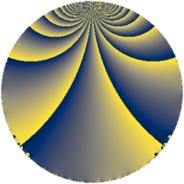# Properties

 Label 195.2.baLevel $195$ Weight $2$ Character orbit 195.ba Rep. character $\chi_{195}(94,\cdot)$ Character field $\Q(\zeta_{6})$ Dimension $24$ Newform subspaces $1$ Sturm bound $56$ Trace bound $0$

# Related objects

## Defining parameters

 Level: $$N$$ $$=$$ $$195 = 3 \cdot 5 \cdot 13$$ Weight: $$k$$ $$=$$ $$2$$ Character orbit: $$[\chi]$$ $$=$$ 195.ba (of order $$6$$ and degree $$2$$) Character conductor: $$\operatorname{cond}(\chi)$$ $$=$$ $$65$$ Character field: $$\Q(\zeta_{6})$$ Newform subspaces: $$1$$ Sturm bound: $$56$$ Trace bound: $$0$$

## Dimensions

The following table gives the dimensions of various subspaces of $$M_{2}(195, [\chi])$$.

Total New Old
Modular forms 64 24 40
Cusp forms 48 24 24
Eisenstein series 16 0 16

## Trace form

 $$24 q + 8 q^{4} + 4 q^{5} + 12 q^{9} + O(q^{10})$$ $$24 q + 8 q^{4} + 4 q^{5} + 12 q^{9} - 4 q^{10} + 4 q^{11} + 24 q^{14} - 2 q^{15} + 16 q^{16} - 16 q^{19} - 16 q^{20} - 8 q^{21} - 16 q^{25} - 48 q^{26} - 12 q^{29} - 4 q^{30} + 8 q^{31} - 32 q^{34} + 10 q^{35} - 8 q^{36} + 8 q^{39} - 48 q^{40} - 40 q^{41} + 40 q^{44} + 2 q^{45} - 24 q^{46} - 16 q^{49} + 20 q^{50} - 24 q^{51} + 20 q^{55} - 24 q^{56} + 12 q^{59} + 48 q^{60} + 20 q^{61} + 48 q^{64} + 14 q^{65} - 56 q^{66} - 8 q^{69} - 56 q^{70} + 4 q^{71} - 12 q^{74} + 16 q^{75} + 8 q^{76} + 136 q^{79} - 4 q^{80} - 12 q^{81} - 16 q^{84} - 4 q^{85} + 48 q^{86} - 64 q^{89} - 8 q^{90} + 60 q^{91} - 48 q^{94} - 28 q^{95} + 40 q^{96} + 8 q^{99} + O(q^{100})$$

## Decomposition of $$S_{2}^{\mathrm{new}}(195, [\chi])$$ into newform subspaces

Label Dim $A$ Field CM Traces $q$-expansion
$a_{2}$ $a_{3}$ $a_{5}$ $a_{7}$
195.2.ba.a $24$ $1.557$ None $$0$$ $$0$$ $$4$$ $$0$$

## Decomposition of $$S_{2}^{\mathrm{old}}(195, [\chi])$$ into lower level spaces

$$S_{2}^{\mathrm{old}}(195, [\chi]) \cong$$ $$S_{2}^{\mathrm{new}}(65, [\chi])$$$$^{\oplus 2}$$